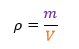# Average Density

Density is a rate of mass per unit volume. So, the average density of an object is its total mass divided by total volume.If you know an item’s density and mass, you can determine its volume. In fact, using algebra, knowing any two parts of the above equation allows you to calculate the third.

Gold particles found in rivers are usually two different substances mixed together. One substance is pure gold alloyed to either silver or copper, mainly. The densities for silver and copper aren’t too different. Copper is about 9 grams per cubic centimeter. While silver is about 10.5 grams per cubic centimeter.

Since a particle of gold is usually the blend of two different metals having different densities, it will have an overall average density somewhere between that of pure gold (19.3 grams per cubic centimeter) and pure copper (9 grams per cubic centimeter). Since this creates a density less than pure gold, the particle has greater volume than if it were pure gold. Grams per cubic millimeter are the units of density in this app. Though density for each metal isn’t specifically displayed on the QuickGoldTally input screen, it is used to calculate output.